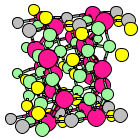Crystal Lattice Structures: Creation Date:  5 May 2002 Last Modified: 21 Oct 2004You can now

• This is, of course, the least symmetry you can get out of a crystal: only translations, no rotations
• Since there is no symmetry whatsoever, we can arbitrarily shift the basis so that one of the atoms is at the origin. In this case, it's the seventh Selenium atom. In the above pictures we've replaced this atom by a Tin atom (colored gray) so that you can see the unit cell boundaries.

• Pearson Symbol: aP16
• Space Group: P1 (Cartesian and lattice coordinate listings available)
• Number: 1
• Reference: Villars and Calvert (Pearson's Handbook), Vol I, p. 1164.
• Primitive Vectors:  A1 = a   X A2 = bx X + by Y A3 = cx X + cy Y + cz Z
• In terms of the traditional lattice parameters: a, b, c, α, β, and γ:  bx = b cos(γ) by = b sin(γ) cx = c cos(β) cy = [b c cos(α) - bx cx]/by cz = [c2 - cx2 - cy2]½
• Basis Vectors:

All the atoms, of course, are on (1a) sites. Atoms 1-4 are As, Atoms 5-8 K, and Atoms 9-16 Se:

 Bi = + x A1 + y A2 + z A3 = + (x a + y bx + z cx) X + (y by + z cy) Y + z cz Z (1a) [i = 1, 2, 3, ... , 16]

Go back to the other structures page.

Go back to Crystal Lattice Structure page.

 Structures indexed by: This is a mirror of an old page created at theNaval Research LaboratoryCenter for Computational Materials ScienceThe maintained successor is hosted at http://www.aflowlib.org/CrystalDatabase/ and published as M. Mehl et al., Comput. Mater. Sci. 136 (Supp.), S1-S828 (2017).